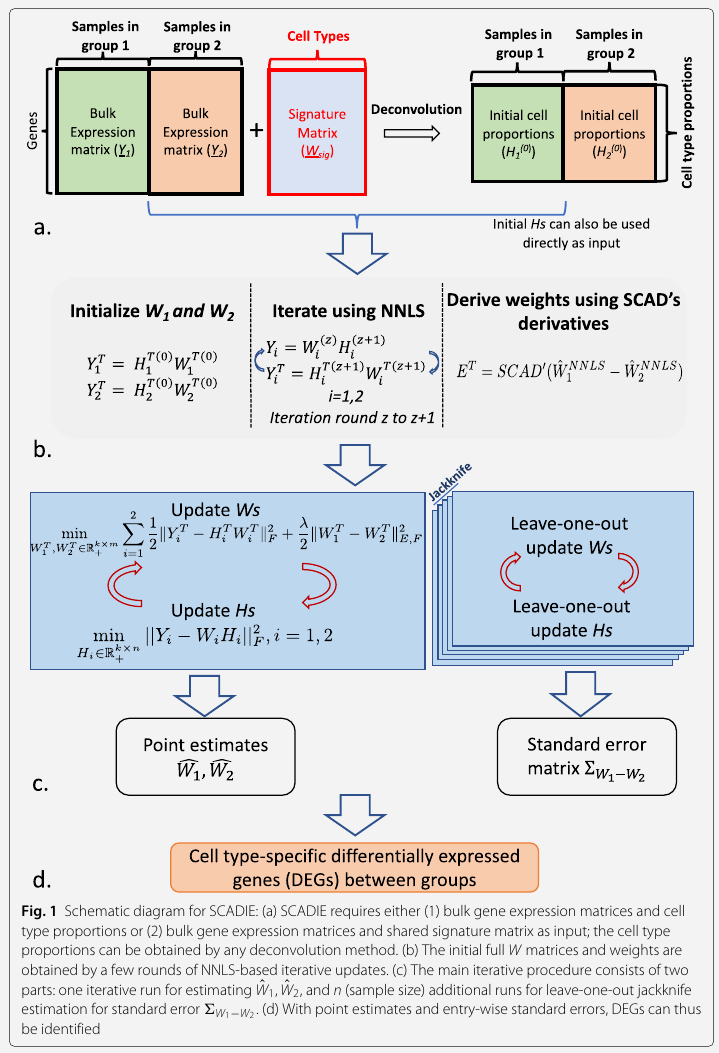# Simultaneous Estimation of Cell Type Proportions and Cell Type-specific Gene Expressions

##### Posted on Oct 12, 2022

simultaneous estimation of cell type proportions and cell type-specific gene expressions using SCAD-based iterative estimating procedure

A challenge in bulk gene differential expression analysis: differentiate changes due to cell type-specific gene expression and cell type proportions

• simultaneously estimates cell type-specific gene expression profiles and cell type proportions
• performs cell type-specific differential expression analysis at the group level
• more accurately identifies cell type-specific differentially expressed genes than existing methods
• robust w.r.t. the choice of deconvolution methods and the sources and quality of input data

Most deconvolution methods assume that the observed bulk gene expression profile is a convex mixture of cell-type specific gene expression profiles. Let $Y$ be the bulk gene expression matrix with $m$ genes and $n$ samples,

$Y = WH, W\in \IR^{(m\times k) +}, H\in \IR^{(k\times n)+}, \text{and } \sum_{i=1}^kH_{ij}=1\forall j\,.$

The principle behind the designs of most existing deconvolution methods is to utilize genes that have distinct expression levels across cell types to infer cell type proportions

• some methods curate a signature matrix with only a subset of cell type-specific genes and gather their expression profiles either from pure cell types or scRNA-seq data
• others use all genes but assign higher weights to genes with more differentiating power to produce a weighted version

Most of downstream analyses were performed under the scheme of single signature matrix, i.e., the same signature matrix was used for different groups of bulk data

The paper claims that a more appropriate model would be that the observed differences in the bulk samples result from not only cell type compositional changes, but also from changes in cell type-specific gene expression profiles.

$Y_1=W_1H_1, Y_2=W_2H_2\,.$Cell type proportions can serve as potential disease predictive biomarkers.

cWAS: cell-type Wide Association Study, integrate genetic data with transcriptomics data to identify cell types whose genetically regulated proportions (GRPs) are disease/trait-associated.

1. build tissue-specific gene expression imputation models using the elastic net
2. with the imputation weights $\hat\beta_{gt}$, obtain the estimation of genetically regulated tissue-level gene expression for gene $g$ in tissue $t$ as $\hat B_{gt} = X_g\hat\beta_{gt}$

Published in categories Note